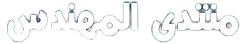# طلب مساعدة في حل سؤالين

``````1-The equation of a circle with radius r
and center located at (a,b) is:

(x-a)^2+(y-b)^2=r^2

Create a MATLAB function to calculate
the points of the circumference of the
circle and plot the resulted xــ coordinates
against y ــ coordinates.

Given the radius r and the
location of the center (a,b).

2- For a circle with radius 5 ,
and center located at (5,5),
use the MATLAB function developed in Question (1),
to return the values of the x and y coordinates.
Plot the circle in an axis of ([-8 8])
in both x and y ــ axis.
``````

تابع هذا الموضوع
http://www.almohandes.org/vb/showthread.php/-46051.html

شكرااااااااااااااااااااااااااااااا

العفو